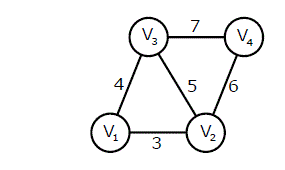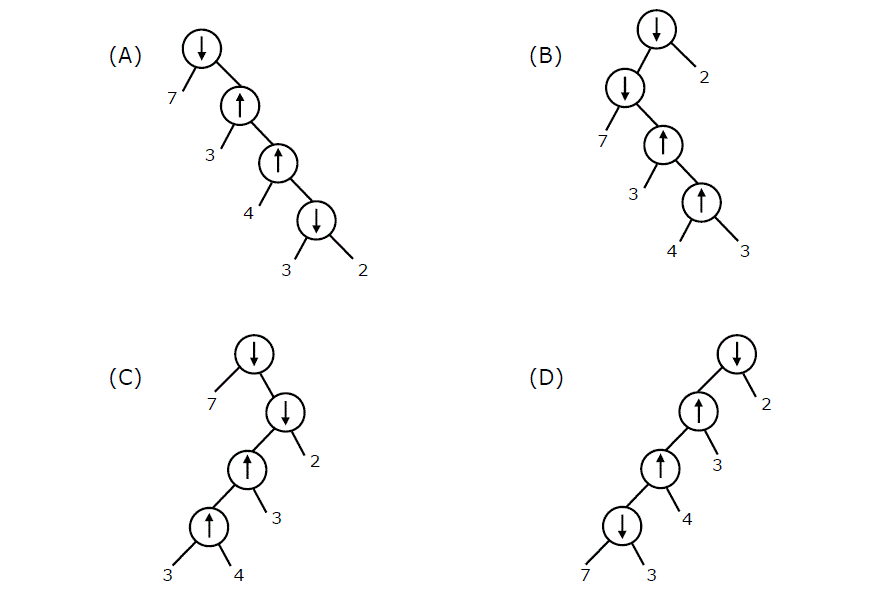# Data Structures and Algorithms | Set 27

Following questions have been asked in GATE CS 2011 exam.

1) An undirected graph G(V, E) contains n ( n > 2 ) nodes named v1 , v2 ,….vn. Two nodes vi , vj are connected if and only if 0 < |i – j| <= 2. Each edge (vi, vj ) is assigned a weight i + j. A sample graph with n = 4 is shown below.What will be the cost of the minimum spanning tree (MST) of such a graph with n nodes?
(A) 1/12(11n^2 – 5n)
(B) n^2 – n + 1
(C) 6n – 11
(D) 2n + 1

Minimum spanning tree for 2 nodes would be

``` (v1) _ (v2)
```

Total weight 3

Minimum spanning tree for 3 nodes would be

``` (v1) _ (v2)
|
(v3)
```

Total weight= 3 + 4 = 7

Minimum spanning tree for 4 nodes would be

``` (v1) _ (v2) _ (v4)
|
(v3)
```

Total weight= 3 + 4 + 6 = 13

Minimum spanning tree for 5 nodes would be

``` (v1) _ (v2) _ (v4)
|
(v3)
|
(v5)
```

Total weight= 3 + 4 + 6 + 8 = 21

Minimum spanning tree for 6 nodes would be

``` (v1) _ (v2) _ (v4) _ (v6)
|
(v3)
|
(v5)
```

Total weight= 3 + 4 + 6 + 8 + 10 = 31

We can observe from above examples that when we add kth node, the weight of spanning tree increases by 2k-2. Let T(n) be the weight of minimum spanning tree. T(n) can be written as

T(n) = T(n-1) + (2n-2) for n > 2
T(1) = 0, T(2) = 0 and T(2) = 3

The recurrence can be written as sum of series (2n – 2) + (2n-4) + (2n-6) + (2n-8) + …. 3 and solution of this recurrence is n^2 – n + 1.

2) The length of the path from v5 to v6 in the MST of previous question with n = 10 is

(A) 11
(B) 25
(C) 31
(D) 41

Any MST which has more than 5 nodes will have the same distance between v5 and v6 as the basic structure of all MSTs (with more than 5 nodes) would be following.

``` (v1) _ (v2) _ (v4) _  (v6) _ .  . (more even numbered nodes)
|
(v3)
|
(v5)
|
.
.
(more odd numbered nodes)
```

Distance between v5 and v6 = 3 + 4 + 6 + 8 + 10 = 31

3) Consider two binary operators ‘↑ ‘ and ‘↓’ with the precedence of operator ↓ being lower than that of the ↑ operator. Operator ↑ is right associative while operator ↓ is left associative. Which one of the following represents the parse tree for expression (7 ↓ 3 ­↑ 4 ­↑ 3 ↓ 2)?Let us consider the given expression (7 ↓ 3 ↑ 4 ↑ 3 ↓ 2).

Since the precedence of ↑ is higher, the sub-expression ([3 ↑ 4 ↑ 3) will be evaluated first. In this sub-expression, 4 ↑ 3 would be evaluated first because ↑ is right to left associative. So the expression is evaluated as ((7 ↓ (3 ↑ (4 ↑ 3))) ↓ 2). Also, note that among the two ↓ operators, first one is evaluated before the second one because the associativity of ↓ is left to right.

Please see GATE Corner for all previous year paper/solutions/explanations, syllabus, important dates, notes, etc.My Personal Notes arrow_drop_up

Article Tags :

Be the First to upvote.

Please write to us at contribute@geeksforgeeks.org to report any issue with the above content.